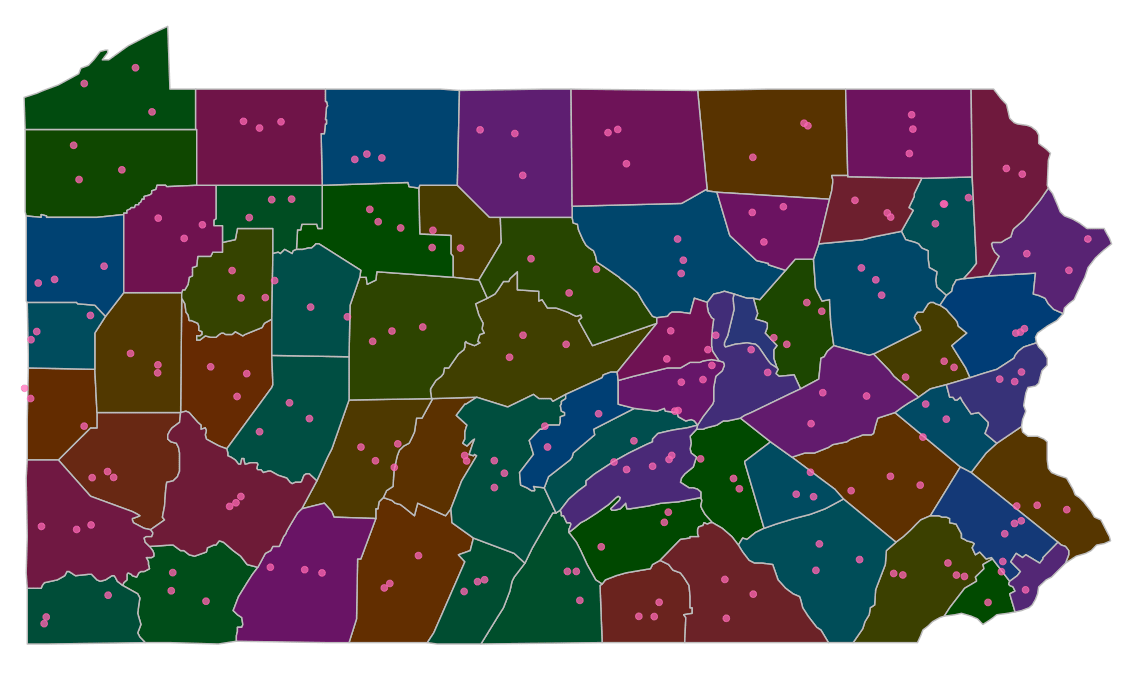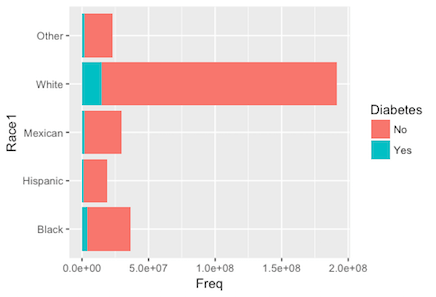Want to share your content on R-bloggers? click here if you have a blog, or here if you don't.### Course Description

You’ve taken a survey (or 1000) before, right? Have you ever wondered what goes into designing a survey and how survey responses are turned into actionable insights? Of course you have! In Analyzing Survey Data in R, you will work with surveys from A to Z, starting with common survey design structures, such as clustering and stratification, and will continue through to visualizing and analyzing survey results. You will model survey data from the National Health and Nutrition Examination Survey using R’s survey and tidyverse packages. Following the course, you will be able to successfully interpret survey results and finally find the answers to life’s burning questions!

### Chapter 1: Introduction to survey data

Our exploration of survey data will begin with survey weights. In this chapter, we will learn what survey weights are and why they are so important in survey data analysis. Another unique feature of survey data are how they were collected via clustering and stratification. We’ll practice specifying and exploring these sampling features for several survey datasets.### Chapter 2: Exploring categorical data

Now that we have a handle of survey weights, we will practice incorporating those weights into our analysis of categorical data in this chapter. We’ll conduct descriptive inference by calculating summary statistics, building summary tables, and constructing bar graphs. For analytic inference, we will learn to run chi-squared tests.### Chapter 3: Exploring quantitative data

Of course not all survey data are categorical and so in this chapter, we will explore analyzing quantitative survey data. We will learn to compute survey-weighted statistics, such as the mean and quantiles. For data visualization, we’ll construct bar-graphs, histograms and density plots. We will close out the chapter by conducting analytic inference with survey-weighted t-tests.### Chapter 4: Modeling quantitative data

To model survey data also requires careful consideration of how the data were collected. We will start our modeling chapter by learning how to incorporate survey weights into scatter plots through aesthetics such as size, color, and transparency. We’ll model the survey data with linear regression and will explore how to incorporate categorical predictors and polynomial terms into our models.